Question

# 20 kN 5 Determine the vertical displacement of joint B. Assume the members are pin connected...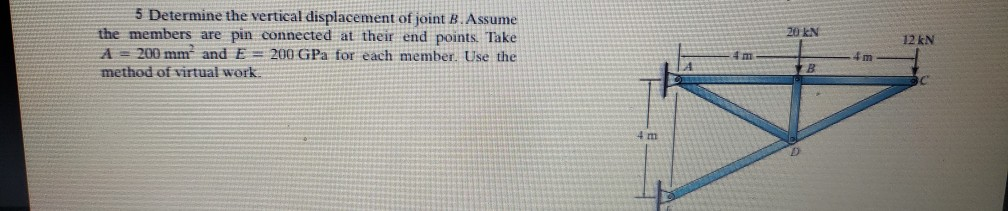20 kN 5 Determine the vertical displacement of joint B. Assume the members are pin connected at their end points. Take A = 200 mm and E= 200 GPa for each member. Use the method of virtual work. 12 kN #m B 4 m

Step 1: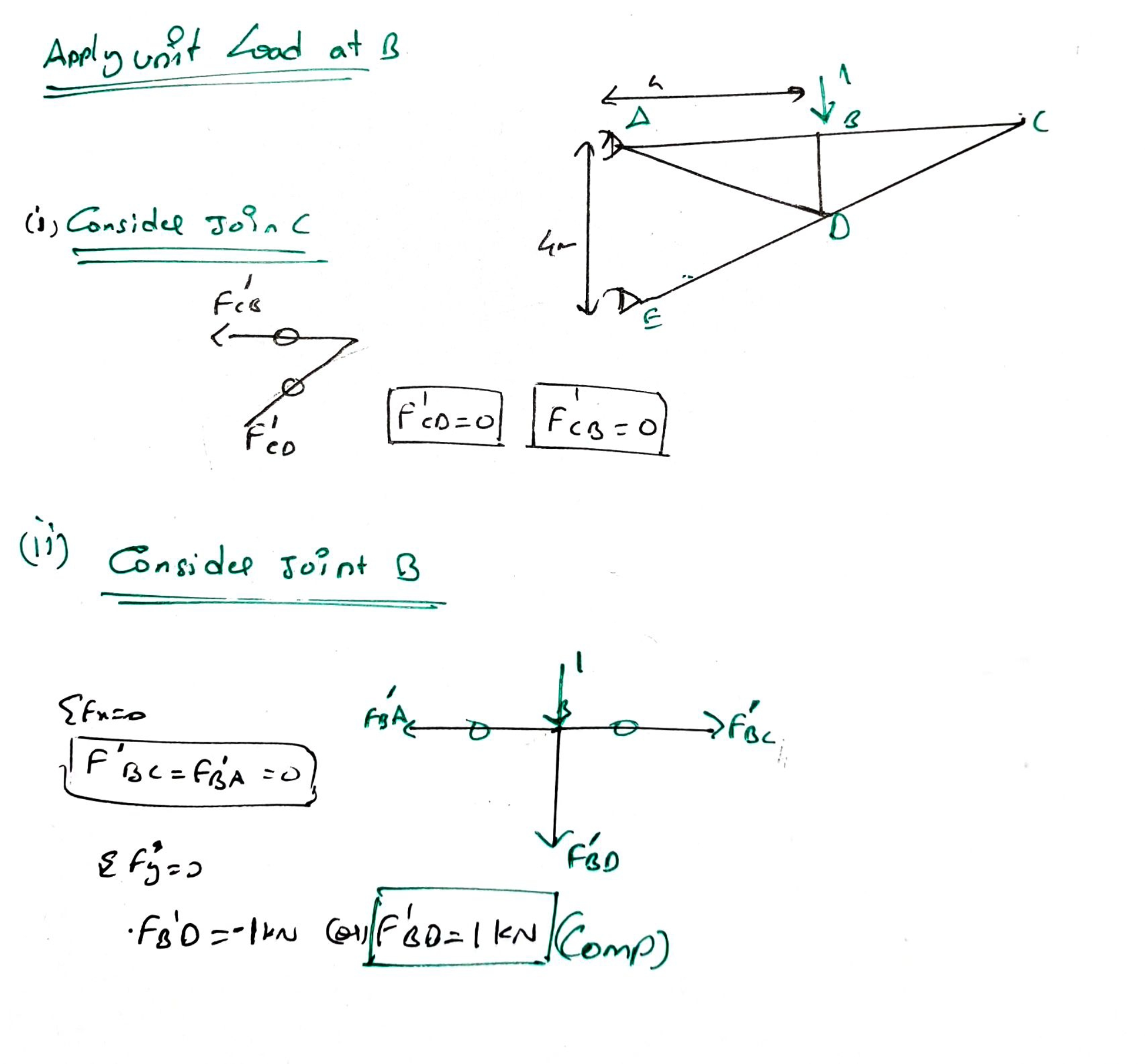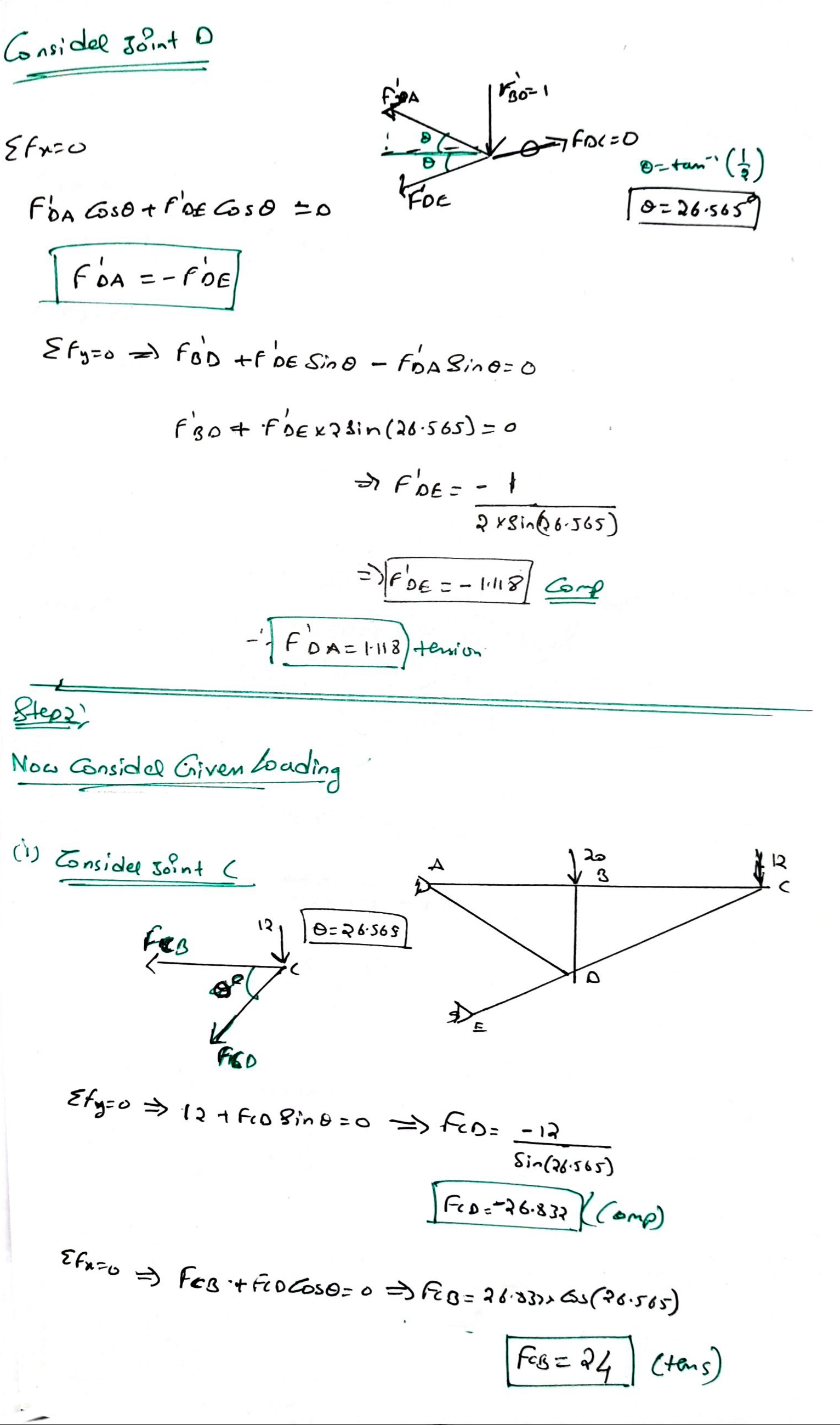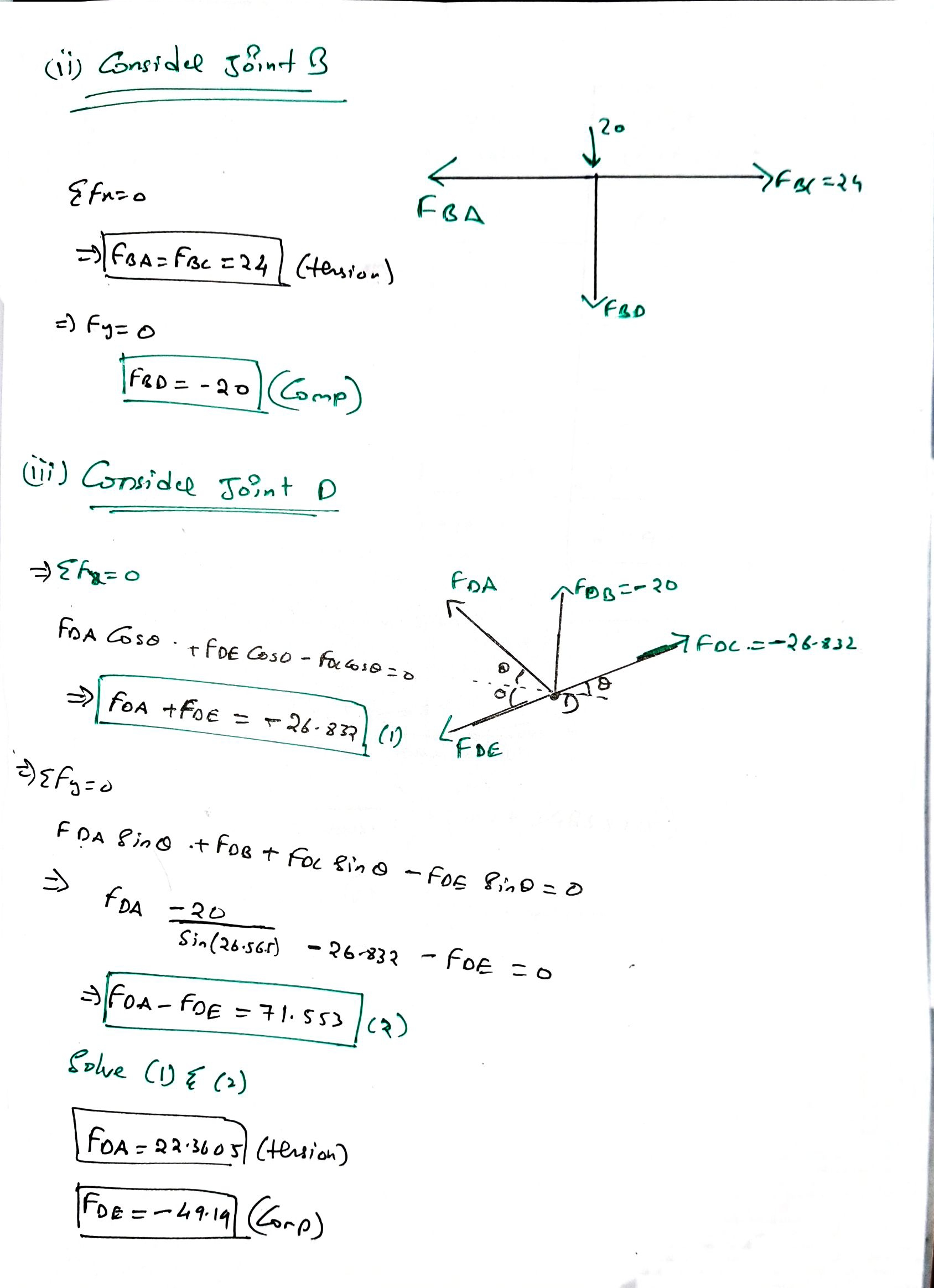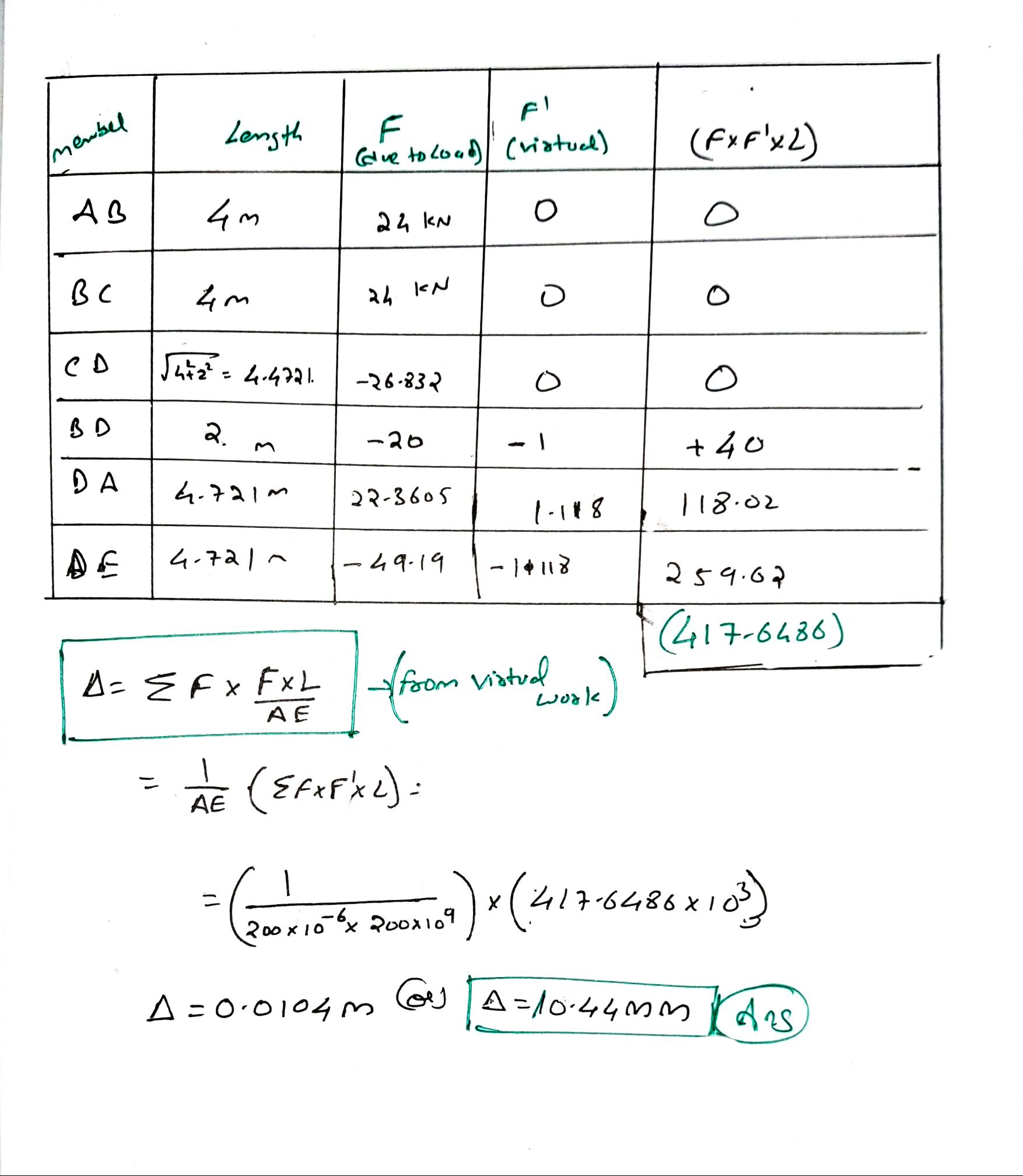Hope this helps

Please feel free to comment on any further queries

All the best :)

#### Earn Coins

Coins can be redeemed for fabulous gifts.

Similar Homework Help Questions
• ### Question 1 (25 points) Determine the horizontal displacement of point C. Assume the members are pin...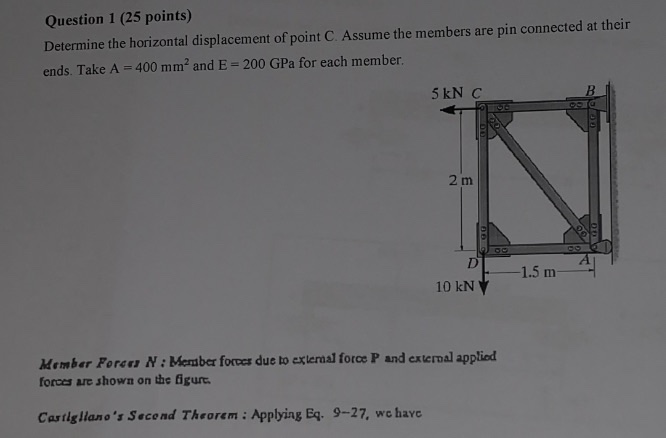Question 1 (25 points) Determine the horizontal displacement of point C. Assume the members are pin connected at their ends. Take A = 400 mm? and E = 200 GPa for each member. 5 kN C 2 m 1.5 m 10 kN Member Foron N: Member forces due to external force P and external applied forces are shown on the figurt. Castigliano's Second Theorem : Applying Eq. 9-27, we have

• ### 2. Determine the vertical displacement of joint D. Use the method of virtual work. AE is...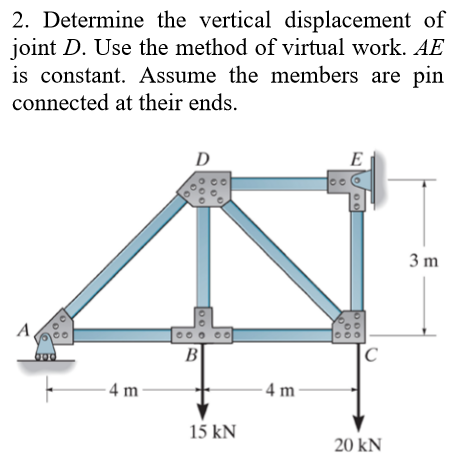2. Determine the vertical displacement of joint D. Use the method of virtual work. AE is constant. Assume the members are pin connected at their ends. 0 0 ooo 0 0 Oo 3 m ВГ –4 m t 15 KN 20 KN

• ### Q3: Determine the vertical and horizontal displacement of joint A for the truss shown in Fig....Q3: Determine the vertical and horizontal displacement of joint A for the truss shown in Fig. (3). each bar is made of steel and has the cross-sectional area of 400mm Take E = 200 GPa Use the method of virtual work. E D 2 m |в -1.5 m -1.5 m 20 KN 40 KN Fig. (3)

• ### Q3: Determine the vertical and horizontal displacement of joint A for the truss shown in Fig....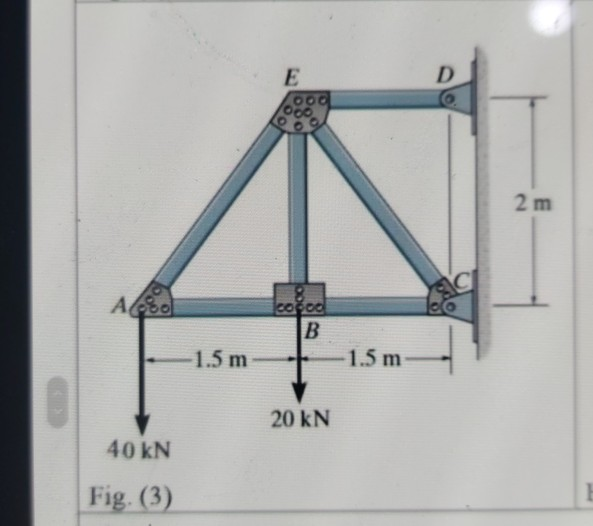Q3: Determine the vertical and horizontal displacement of joint A for the truss shown in Fig. (3), each bar is made of steel and has the cross-sectional area of 400mm?. Take E = 200 GPa. Use the method of virtual work. E D 00. 2 m СІ Ao cocoa B 1.5 m 1.5 m 20 KN 40 kN Fig. (3)

• ### stion 1 Determine the total vertical displacement of joint A (due to the load and tempreature...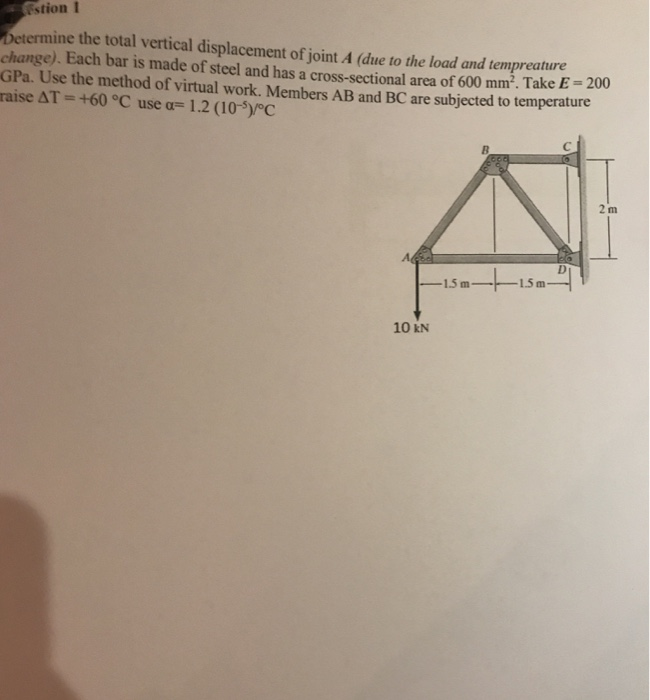stion 1 Determine the total vertical displacement of joint A (due to the load and tempreature change). Each bar is made of steel and has a cross-sectional area of 600 mm². Take E - 2001 GPa. Use the method of virtual work. Members AB and BC are subjected to temperature raise AT = +60 °C use a= 1.2 (10-5)/°C —15 m- 1.5m 10 KN

• ### Part A Consider the truss shown in (Figure 1). Assume the members are pin connected at...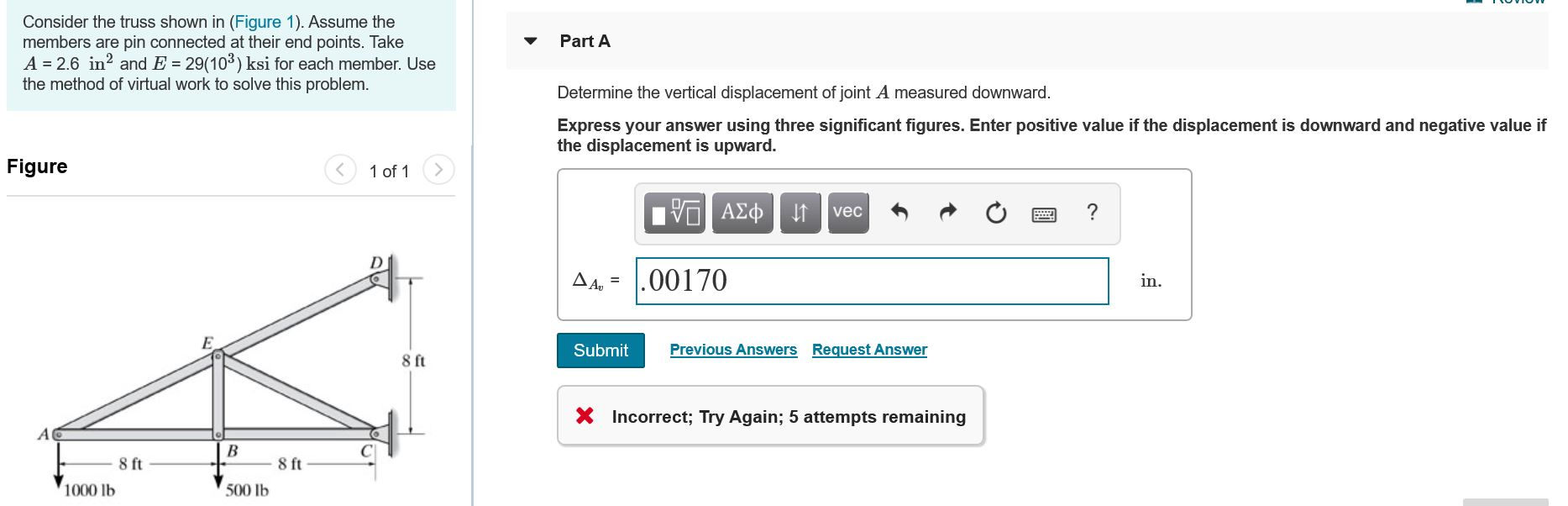Part A Consider the truss shown in (Figure 1). Assume the members are pin connected at their end points. Take A = 2.6 in? and E = 29(103) ksi for each member. Use the method of virtual work to solve this problem. Determine the vertical displacement of joint A measured downward. Express your answer using three significant figures. Enter positive value if the displacement is downward and negative value if the displacement is upward. Figure < 1 of 1 V...

• ### 2. Determine the vertical displacement at joint B and horizontal displacement at joint D using Castigliano's...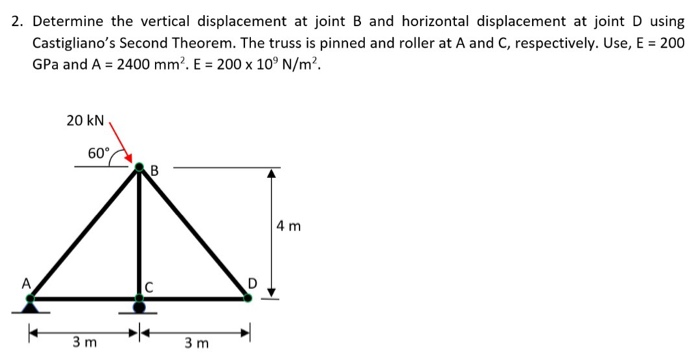2. Determine the vertical displacement at joint B and horizontal displacement at joint D using Castigliano's Second Theorem. The truss is pinned and roller at A and C, respectively. Use, E = 200 GPa and A = 2400 mm. E = 200 x 10°N/m². 20 KN 60 CAB 4 m 3m 3m

• ### Solve It fast Please Q3: Determine the vertical and horizontal displacement of joint A for the...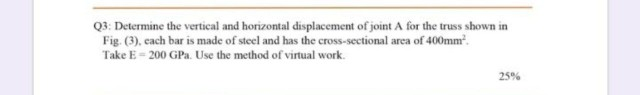Solve It fast Please Q3: Determine the vertical and horizontal displacement of joint A for the truss shown in Fig. (3). cach bar is made of steel and has the cross-sectional area of 400mmº. Take E = 200 GP. Use the method of virtual work. 25% E OO D 2 m AAS ered B -1.5 m -1.5 m 20 KN 40 KN Fig. (3)

• ### i need the answer quickly fast as you can Q3: Determine the vertical and horizontal displacement...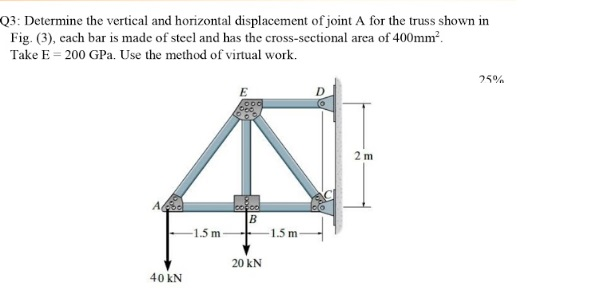i need the answer quickly fast as you can Q3: Determine the vertical and horizontal displacement of joint A for the truss shown in Fig. (3), cach bar is made of steel and has the cross-sectional area of 400mm? Take E = 200 GPa. Use the method of virtual work. 25% E 2 m А. cool B 1.5 m -1.5 m 20 KN 40 KN

• ### Determine the horizontal displacement at A. Take E = 200 GPa. The moment of inertia of...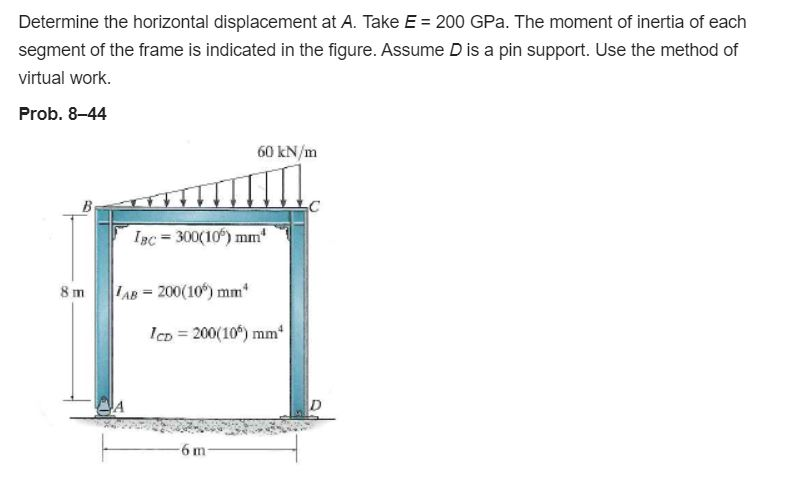Determine the horizontal displacement at A. Take E = 200 GPa. The moment of inertia of each segment of the frame is indicated in the figure. Assume D is a pin support. Use the method of virtual work. Prob. 8-44 60 kN/m B 1pc = 300(10%) mm 8 m AB= 200(106) mm IcD = 200(10%) mm 6 m